Reach Us+44-1522-440391
Observables and unobservables in a non-associative quantum theory | OMICS International
Journal of Generalized Lie Theory and Applications
All submissions of the EM system will be redirected to Online Manuscript Submission System. Authors are requested to submit articles directly to Online Manuscript Submission System of respective journal.

# Observables and unobservables in a non-associative quantum theory

Vladimir DZHUNUSHALIEV*

Deptartment of Physical and and Microelectronical Engineering,Kyrgyz-Russian Slavic University, Kievskaya Str. 44, 720021 Bishkek, Kyrgyz Republic

*Corresponding Author:
Vladimir DZHUNUSHALIEV 1
Deptartment of Physical and and Microelectronical Engineering
Kyrgyz-Russian Slavic University Kievskaya
Str. 44, 720021 Bishkek, Kyrgyz Republic
E-mail: [email protected]

Received Date: September 06, 2007; Revised Date: January 31, 2008

Visit for more related articles at Journal of Generalized Lie Theory and Applications

#### Abstract

It is shown that the non-associative operators in a non-associative quantum theory are unobservables. The observable quantity may be presented only by the elements of some asso- ciative subalgebra. It is shown that the elements of the associative subalgebra are extended objects that can be similar to strings. It is assumed that the non-associative quantum eld theory can be applied to the quantization of strongly interacting elds. The method for obtaining eld equations in a non-associative case is given.

#### Introduction

Non-associativity in physics is a very seldom visitor. In  the attempt was made to obtain a possible generalization of quantum mechanics on any numbers including non-associative numbers: octonions. In  the author applies non-associative algebras to physics. This book covers topics ranging from algebras of observables in quantum mechanics, to angular momentum and octo- nions, division algebras, triple-linear products and Yang - Baxter equations. The non-associative gauge theoretic reformulation of Einstein's general relativity theory is also discussed. In  one can nd the review of mathematical de nitions and physical applications for the octonions. The modern applications of the non-associativity in physics are: in [4,5] it is shown that the require- ment that nite translations be associative leads to Dirac's monopole quantization condition; in [6,7] Dirac's operator and Maxwell's equations are derived in the algebra of split-octonions. In this paper we would like to show that the application of the non-associativity in quantum theory leads to the interesting fact: the appearance of extended particles which are similar to strings in string theory.

#### Unobservables in a non-associative quantum theory

The observability of a physical quantity M in quantum mechanics means that it is presented as an operator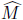with the following properties:

• The eigenvalues of the operator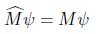(2.1)

gives us the possible spectrum of M values.

• The averaged value of the physical quantity is given as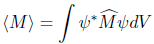(2.2)

• The values M from (2.1) and hMi from (2.2) are real numbers.

The same is valid for quantum eld theory with some complexi cations. Now we would like to show that the non-associativity does not allow us to find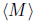and M as real numbers. The proofs thatand M are real numbers are well known in quantum mechanics. At the end of the proof that M is a real number we have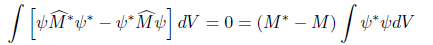(2.3)

and immediately we see that M = M*. The crucial point here is that on the LHS of Eq. (2.3) we have the triple products like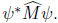In the quantum nonassociative theory this product depends on the rearrangements of brackets and the LHS of Eq. (2.3) may thus be nonzero. The situation for the proof that the averaged valueis a real number is similar.

The main result of this simple consideration is: non-associative operators present unobservable quantities.

At the rst sight this statement destroys any attempt to give any physical sense to a non- associative quantum theory. Nevertheless the outlet exists: if the non-associative algebra of quantum eld operators has an associative subalgebra then these associative operators are ob- servables. This observation leads to the remarkable result: the observables in a non-associative quantum eld theory is a product of nonobservable quantities.

#### Observables in a non-associative quantum eld theory

According to the previous remarks, let us consider an observable quantity φ in a hypothesized non-associative quantum eld theory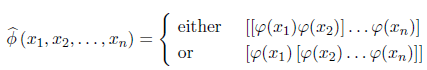(3.1)

here xi are the Minkowskian coordinates;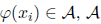is a non-associative algebra of quantum operators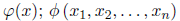is an element of an associative subalgebra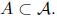Now we would like to consider the physical sense of Eq. (3.1). For simplicity we will consider the product of two non-associative operators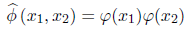(3.2)

It is necessary to mention that the decomposition (3.2) is very similar to slave-boson decom- position in t - J model of High-Tc superconductivity (for a review, see ) and spin-charge separation in the non-Abelian gauge theories  - . Let us consider the (anti)commutator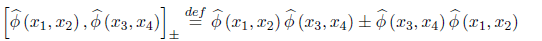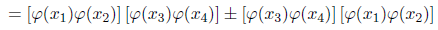(3.3)

Using (±) associators (which are yet unknown)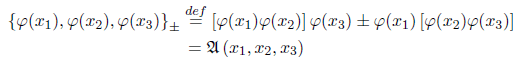(3.4)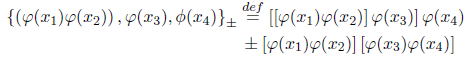(3.5)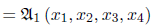(3.6)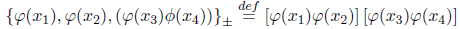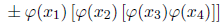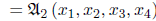(3.7)

we can calculate the RHS of Eq. (3.3)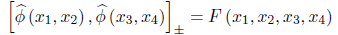(3.8)

Here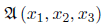and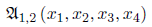are some combinations of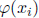operators and real functions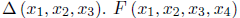is an associative operator but probably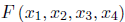is a real function of coordinates xi. It is very important to emphasize that the object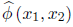not decomposable, i.e. we can not observe its componentsbecause we have shown above that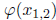are unobservable quantities. We only can observe the whole object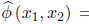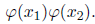Now we would like to compare this situation with the propagator of string in string theory. In string theory the propagator is a Veneziano amplitude that is the function of four coordinates (or four impulses in the momentum space). Comparing Eq. (3.8) with the calculation of a string propagator we can offer the idea that the RHS of (3.8) is the propagator of an extended object which can be a string if the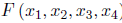is equal to the Veneziano amplitude. The big di erence between string in string theory and extended object in a non-associative quantum eld theory is that in the rst case the string coordinates are observable quantities but in the second case the inner structure of a non-associative extended object is unobservable.

#### Field equations in a non-associative quantum eld theory

In the approach to the quantization of strongly interacting elds presented above we assume that any operator of strongly interacting fields can be presented as the product that is the generalization of slave-boson decomposition in t - J model of High-Tc superconductivity  and spin-charge separation in the non-Abelian gauge theories  - . The non-associative factors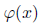are distributed in the spacetime and should have dynamical equations de ning such distribution. Thus the question arises: what kind of eld equations describe the dynamics of non-associative operators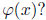Our point of view is that the corresponding equations simply are the eld equations of the associative eld operators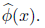Then all derivatives can be calculated for the non-associative operator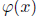and in the result we deduce the eld equations for the non-associative eld operatorsLet us, for example, consider non-Abelian gauge theory. In this case we have the decomposi- tion (3.1) (which in nothing else but the generalization of spin-charge separation  - ). The eld equations are the Yang-Mills equations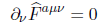(4.1)

here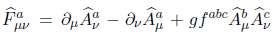is the eld strength operator;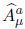is the operator of gauge eld; a = 1; 2; : : : ; n is the color index for SU(n) gauge eld; fabc are the SU(n) struc-tural constants and u;v = 0; 1; 2; 3 are spacetime indices. The operator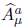has the following decomposition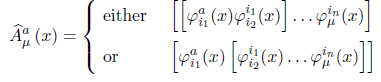(4.2)

where i1; i2; : : : ; in are inner indexes similar to one in spin-charge separation  - . Comparing with the decomposition(3.1) the decomposition (4.2) is given at one point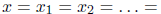xn. Inserting the decomposition (4.2) in the eld strength operator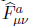and afterwards in the Yang-Mills equations (4.1) we shall receive equations for the nonassociative operator φ(x).

#### Outlook

To summarize we have shown that if a non-associative algebra of quantum eld operators has an associative subalgebra, then the operator of extended particles can be represented similarly to the string representation of elementary particles in string theory.

It is necessary to note that the decomposition (3.1) can be thought of as a variation of the idea about hidden variables in the theory of hidden parameters.

#### Acknowledgments

Author acknowledges D. Singleton for the invitation to do research at Fresno State University and the support of a CSU Fresno Provost Award Grant.

#### References

Select your language of interest to view the total content in your interested language

### Article Usage

• Total views: 12045
• [From(publication date):
November-2008 - Aug 23, 2019]
• Breakdown by view type
• HTML page views : 8235
• PDF downloads : 3810

## Post your commentCan't read the image? click here to refresh
###### Peer Reviewed Journals

Make the best use of Scientific Research and information from our 700 + peer reviewed, Open Access Journals

International Conferences 2019-20

Meet Inspiring Speakers and Experts at our 3000+ Global Annual Meetings

Top